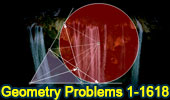# Online Math: List of Go Geometry Problems Collection

 Go Geometry Problems Page 1Open Geometry Problems Problems      Ten Geometry Problems      Visual Index   Parallel lines   Perpendicular lines    Collinear Points   Triangle   Metric Relations   Quadrilateral    Triangle & Squares   Polygons    Pentagons    Hexagons    Heptagons    Octagons   Circle   Triangle Centers   Congruence   Similarity, Ratios, Proportions Dynamic Geometry 1481. Five Tangential or Circumscribed Quadrilaterals, Pitot Theorem, Congruence, Step-by-step Illustration. GeoGebra, iPad. Dynamic Geometry 1480. Japanese Theorem for Cyclic Polygon, Sangaku, Triangulation, Non-intersecting Diagonals, Sum of Inradii, Invariant, Step-by-step Illustration. GeoGebra, iPad. Dynamic Geometry 1479. Triangle, Circumcircle, Angle Bisector, Perpendicular Bisector, Chord, Concyclic Points, Parallel Lines,, Step-by-step Illustration. GeoGebra, iPad. Dynamic Geometry 1478. Cyclocevian, Reuschle-Terquem Theorem, Concurrent Cevians, Triangle, Circumcircle, Secant line, Step-by-step Illustration. GeoGebra, iPad. Dynamic Geometry 1477. Miquel's Pentagram Theorem, Pentagon, Triangle, Circumcircles, Concyclic Points, Step-by-step Illustration. GeoGebra, iPad. Dynamic Geometry 1476. Droz-Farny Line Theorem, Triangle, Orthocenter, Perpendicular, Collinear Midpoints, Step-by-step Illustration. GeoGebra, iPad. Dynamic Geometry 1475. Clifford Intersecting Circles Theorem, Step-by-step Illustration, GeoGebra, iPad. Dynamic Geometry 1473. Kosnita's Theorem, Triangle, Four Circumcenters, Concurrent Line, Step-by-step Illustration. Dynamic Geometry 1472. Cyclic Quadrilateral, Perpendicular Diagonals, Rectangle, Step-by-step Illustration. Dynamic Geometry 1471. Equilateral Triangle, Inside/Outside Point, Incenters, Tangency Points, Concurrent Lines, Step-by-step Illustration. Dynamic Geometry 1470. Tangential Quadrilateral, Incircles, Tangent, Parallel, Rhombus, Step-by-step Illustration. Dynamic Geometry 1469. Triangle, Circumradius, Inradius, Midpoints, Arcs, Sum of Distances, Step-by-step Illustration. Dynamic Geometry 1468. Steiner's Theorem, Triangle, Circumradius, Inradius, Sum of Exradii, Step-by-step Illustration. Geometry Problem 1467. Square, Rectangle, Triangle, Area. Geometry Problem 1466. Tangential Quadrilateral, Newton Line, Incenter, Midpoint, Diagonal, Collinear Points. Step-by-step Illustration using GeoGebra. Geometry Problem 1465. Tangential Quadrilateral, Incenter, Inscribed Circle, Equal Sum of Areas. Step-by-step Illustration using GeoGebra. Geometry Problem 1464. Quadrilateral, Interior Point, Midpoint of Sides, Equal Sum of Areas. Step-by-step Illustration using GeoGebra. Geometry Problem 1463. Parallelogram, Interior Point, Opposite Triangles with Equal Sum of Areas. Step-by-step Illustration using GeoGebra. Geometry Problem 1462. Newton-Line, Newton-Gauss Line, Complete Quadrilateral, Midpoints of Sides and Diagonals, Collinear Points. Geometry Problem 1461. Quadrilateral, Triangle, Angles, 30-60 Degree, Congruence, Auxiliary Lines. Dynamic Geometry 1460. Newton-Gauss Line, Complete Quadrilateral, Midpoints of Diagonals, Collinear Points, Step-by-step Illustration using GeoGebra. Geometry Problem 1459. Two Triangles, Orthocenter, Midpoint, Perpendicular, Step-by-step Illustration using GeoGebra. Geometry Problem 1458. Triangle, Incircles, Excircle, Area, Step-by-step Illustration using GeoGebra. Geometry Problem 1457. Altitudes, Circles, Similarity, Product of the Inradii Lengths, iPad. Step-by-step illustration using GeoGebra. Geometry Problem 1456. Altitudes, Orthic Triangle, Circumcircle, Parallel lines, Similarity, Area, iPad. Step-by-step illustration using GeoGebra. Geometry Problem 1455. Nagel Point, Excircles, Incircle, Congruent Segments, iPad. Step-by-step illustration using GeoGebra. Geometry Problem 1454. Intersecting Circles, Perpendicular Lines, Cyclic Quadrilateral, Collinear Points, iPad. Step-by-step illustration using GeoGebra. Geometry Problem 1453. Two Semicircles, Cyclic Quadrilateral, Concyclic Points, iPad. Dynamic Geometry 1452. Japanese Theorem, Sangaku, Cyclic Quadrilateral, Incenter, Rectangle, Inradius. Step-by-step illustration using GeoGebra. Dynamic Geometry 1451. Orthopole of a Line. Step-by-step illustration using GeoGebra. Dynamic Geometry 1450. Ortholine, Steiner Line, Complete Quadrilateral, Collineal Orthocenters. Step-by-step illustration using GeoGebra. Geometry Problem 1400.Triangle with three rectangles on the sides, Perpendicular Bisectors, Concurrency. Geometry Problem 1391.Two Triangles, Ratio of Areas, Circumcircle. Geometry Problem 1381.Parallelogram, Exterior Point, Triangle, Area. Geometry Problem 1361. Triangle, Two Nine-Point Circles, Feuerbach's Circle, Euler's Circle, Congruent Angles, Sketch, iPad Apps. Geometry Problem 1200 Square, Right Triangle, Perpendicular, 90 Degrees, Equal Areas. Geometry Problem 900 Intersecting Circles, Common External Tangent, Secant, Angle, Congruence. GeoGebra, HTML5 Animation for Tablets. Solution. Geometry Problem 800 de Gua's Theorem, Pythagorean theorem in 3-D, Tetrahedron, Cubic Vertex, Triangular Pyramid, Apex, Height, Right Triangle Area, Base Area, Projected Area. Solution. Geometry Problem 700. Equilateral Triangle, Circle, Circular Segment, Midpoint of a side, Metric Relations. Solution. Geometry Problem 600 Circle, Area, Tangent, Semicircle, Tangency Point. Solution. Geometry Problem 500. Circle, Diameter, Chord, Perpendicular, Measure. Solution. Geometry Problem 400. Triangle, Angle bisector, Circumcircle, Perpendicular, Congruence. Solution. Geometry Problem 300. Tangents to a circle, Secants, Square. Solution. Geometry Problem 200. RightTriangle, Incircle, Excircles, Points of Tangency, Inradius. Solution. Geometry Problem 100. Circle Area, Archimedes' Book of Lemmas. Solution. Geometry Problem 1. Triangle, median, angles, 45 degrees. Solution Go to Page: Previous | 1 | 2 | 3 | 4 | 5 | 6 | 7 | 8 | 9 | 10 | 11 | 12 | 13 | 14 | 15 | Next

Home | Geometry | Problems | Visual Index | Problem Art | GalleryEmail
Last updated: May 24, 2019